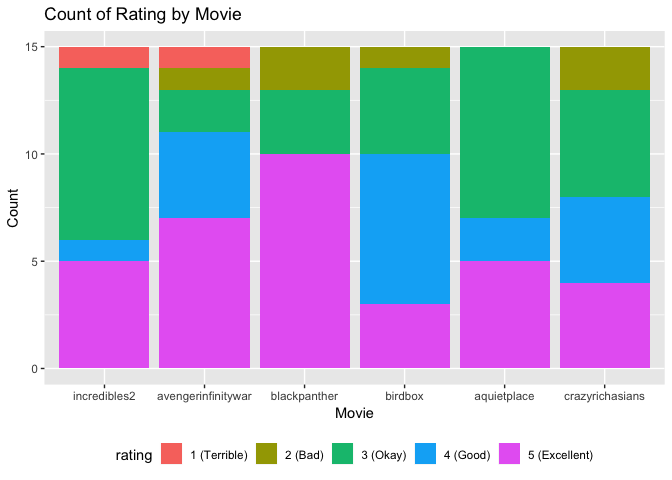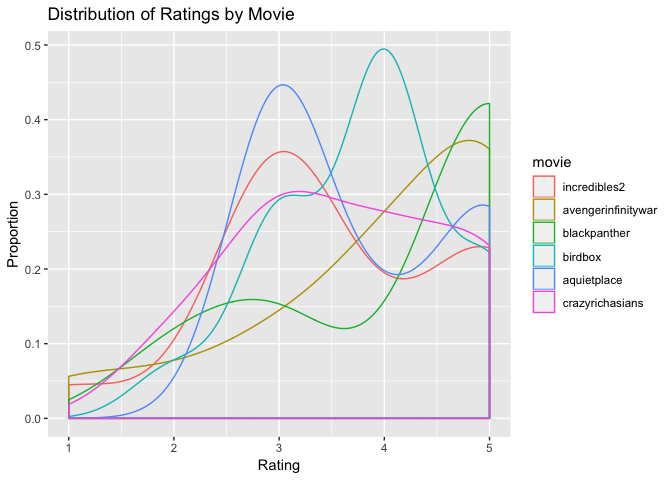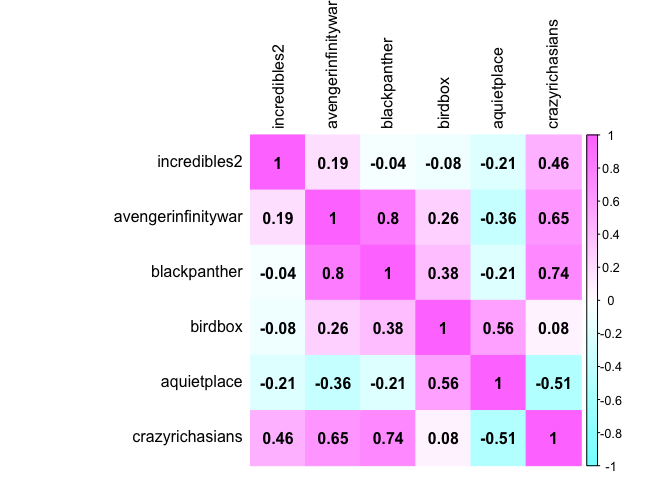# Assignment 2: Movie Dataset

### Introduction

For this assignment, 15 people were surveyed about six recent movies. The movies included in the survey are:

• Incredibles 2
• Avengers: Infinity War
• Black Panther
• Birdbox
• A Quiet Place
• Crazy Rich Asians

The results of this survey were collected using Google Forms and participants were asked to rate each movie using the following scale:

• 5 (Excellent)
• 4 (Good)
• 3 (Okay)
• 1 (Terrible)

The libraries that will be used for this assignment are RpostgreSQL, ggplot2, plyr, reshape, corrplot, and dt.

Use the RPostgreSQL library to load the SQL Server.

drv <- dbDriver("PostgreSQL")
con <- dbConnect(drv,
dbname = "movies",
host="localhost",
user="use",
password="password");

### Load Movies Dataframe from SQL Database

Load and query the movies database from SQL. The SQL query is located inside this github repository. The default movies dataframe is printed below.

movies_default <- dbGetQuery(con,
statement = paste('SELECT * FROM movies'));
datatable(movies_default,
class = 'cell-border stripe',
options = list(
columnDefs = list(list(className = 'dt-center', targets ="_all"))))

### Tranform Movies Dataframe

The format of the current dataframe is not useful for plotting (although it is ideal for correlation plots). We transform the dataframe using the reshape package - this gives each row a unique id-variable combination.

movies_default$personid <- (1:nrow(movies_default)) movies <-melt(movies_default, id=c("personid")) colnames(movies) <- c("personid", "movie", "rating") datatable(movies, class = 'cell-border stripe', rownames = FALSE, options = list( columnDefs = list( list(className = 'dt-center', targets ="_all")))) #### Plotting Ordinal Variables The movie ratings are ordinal variables, so we can use the factor function to encode ratings as a factor. In order to plot ratings, we create another vector that only contains the numeric movie rating. movies$rating <- factor(movies$rating, levels = rev(c("5 (Excellent)", "4 (Good)", "3 (Okay)", "2 (Bad)", "1 (Terrible)"))) levels(movies$rating)
##  "1 (Terrible)"  "2 (Bad)"       "3 (Okay)"      "4 (Good)"
##  "5 (Excellent)"
movies$ratingnum <- as.numeric(substr(movies$rating, 1, 1))

### Movie Rating Summaries

First, we can summarize the movie ratings using mean and median centrality measures.

meantable <- setNames(aggregate(movies$ratingnum, list(movies$movie), mean),
c("movie","mean"))
mediantable <- setNames(aggregate(movies$ratingnum, list(movies$movie), median),
c("movie","median"))
datatable(merge(x = meantable, y = mediantable,
by = "movie", all.x = TRUE))

### Visualizing Movie Ratings

Movie Rating Counts

The table below displays the amount of each category of ratings by movie. We can see that the perception of all the movies is generally positive or neutral. Black Panther has the highest count of excellent ratings, followed by Avengers: Infinity War.

ggplot(movies, aes(x=movie, fill= rating)) +
geom_bar() +
theme(legend.position="bottom") +
labs(title ="Count of Rating by Movie", x = "Movie", y = "Count")Rating Distributions

This next table shows the distribution of rating categories for each movie. We can see the following movies have the highest proportion of okay ratings:

• A Quiet Place
• Incredibles 2
• Crazy Rich Asians

Bird Box had the highest proportion of good ratings, and most surveyors rated Black Panther and Avengers: Infinity War as excellent.

ggplot(movies, aes(x=ratingnum, color=movie)) +
geom_density() +
labs(title ="Distribution of Ratings by Movie",
x = "Rating",
y = "Proportion")### Recommending Movies

Based on the survey responses, how do we determine whether someone will enjoy one of these movies? Using the correlation plot below, we can make recommendations using movies that a person has already watched and enjoyed.

For example:

• If someone enjoyed Avengers: Infinity War, they are likely to enjoy Black Panther
• If someone enjoyed Black Panther, they are likely to enjoy Crazy Rich Asians
• If someone enjoyed Bird Box, they may also enjoy A Quiet Place
movies_default\$personid <- NULL
for(x in names(movies_default)){
movies_default[[x]] <- as.numeric(substr(movies_default[[x]], 1, 1))
}
moviescor <- cor(movies_default)
corrplot(moviescor,
method="color",
col = cm.colors(100))